# Comment on ‘Conditional estimation and inference to address observed covariate imbalance in randomized clinical trials’

Thanks to Tim Morris for letting me know about a paper just published in the journal Clinical Trials by Zhang et al, titled ‘Conditional estimation and inference to address observed covariate imbalance in randomized clinical trials’. Zhang et al propose so called conditional estimation and inference to address observed covariate imbalance in randomised trials. They introduce the setup of randomised trials with covariates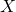, randomised treatment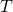, and outcome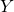. They begin with a framework that treats all three as random in repeated sampling, and review the unadjusted estimator of the marginal mean difference in outcome, and a covariate adjusted estimator based on earlier work by Tsiatis and others.

For covariate adjusted regression analysis of randomised trials with simple randomisation, an argument based on ancillarity justifies performing inference for the parameters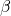in the regression ofonandas ifandare fixed even if in truth they are random in repeated samples.

Zhang et al target the marginal mean difference effect. In a linear model with main effects ofand, this is estimated by the coefficient of treatment in the model, and hence we can base inferences on the conditional standard errors from the linear model due to the aforementioned ancillarity argument. More generally though, the marginal mean difference depends not only on, but also on the marginal distribution ofin the (super) population. The impact of this on the repeated sampling variance is seen in the final equation of page 5 of Zhang et al, where they note that the marginal estimate of variance is equal to the conditional one plus an additional term. From this expression, one can see that in the linear model case with main effects ofand, the first term in equation 5 is zero, and thus the marginal and conditional variance estimators are identical. More generally though, this is not true. This mirrors the situation when one is interested in estimating the marginal mean under each treatment, as I have written about before.

Zhang et al state on page 5 that because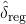is motivated by a possible conditional bias, it is only appropriate that the associated inferences be made conditionally on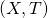‘. I don’t really buy this argument, especially since, as Zhang et al themselves note, their estimatoris the same as the one previously developed by Tsiatis and others from the perspective of minimizing variance whenare random. They are just two ways of looking at the same thing.

The first simulation study Zhang et al perform keepsfixed in repeated samples, and examines the performance of their conditional inference estimators. As one would expect, an unadjusted treatment effect estimator here is biased because there is a persistent imbalance (i.e. confounding) betweenandsinceare being held fixed at some values. In contrast the covariate adjusted estimator is unbiased, the conditional variance estimator is unbiased for the repeated sampling variance of. A question however is whether this simulation is relevant in any way to the real world? If we were to repeat trials, unless the covariates are fixed by design (and if they were you wouldn’t fix them to be imbalanced),would vary randomly in repeated trials. Thus I do not see why the conditional variance is appropriate.

Their second simulation study allowsto be random in repeated trials. As they note on page 8, the conditional variance estimator is expected to underestimate the marginal variance of, but the difference in their simulation is very small. They then state that The Wald confidence interval based onand its conditional standard error is expected to produce (nearly) correct coverage, conditionally and hence marginally, which is confirmed in Table 4.’ I am not sure why they make this claim, particularly in light of the preceding statement that the conditional variance estimator will underestimate the true marginal variance of the adjusted estimator.

Below we perform a simulation study to demonstrate that valid inference for the marginal mean difference in a non-linear model whenare not fixed in repeated samples requires the use of the marginal, not conditional variance estimator. From the expression for the marginal variance estimator given by Zhang et al, we can see that the difference will be larger when there is more variability in thespecific predicted mean differences from the marginal mean difference. To make this larger, we will include an interaction betweenandin the model. The R code for the simulation is as follows:

library(MASS)

expit <- function(x) {
exp(x)/(1+exp(x))
}

nSim <- 100000
n <- 1000

deltahatreg <- array(0, dim=c(nSim))
condVar <- array(0, dim=c(nSim))
margVar <- array(0, dim=c(nSim))

set.seed(6722)

for (i in 1:nSim) {
#print(i)

x <- rnorm(n)
z <- 1*(runif(n)<0.5)
xz <- x*z

xb <- x+1*z-2*xz

y <- 1*(runif(n) < expit(xb))

simdata <- data.frame(y,x,z)

mod <- glm(y~x+xz+z, family="binomial")

activePredxb <- cbind(rep(1,n),x,x,rep(1,n)) %*% coef(mod)
activePred <- expit(activePredxb)
controlPredxb <- cbind(rep(1,n),x,rep(0,n),rep(0,n)) %*% coef(mod)
controlPred <- expit(controlPredxb)
deltahatreg[i] <- mean(activePred)-mean(controlPred)

#conditional variance
outer <- colMeans(as.numeric(expit(activePredxb)*(1-expit(activePredxb))) * cbind(1,x,x,rep(1,n))) - colMeans(as.numeric(expit(controlPredxb)*(1-expit(controlPredxb))) * cbind(1,x,rep(0,n),rep(0,n)))
outer <- array(outer, dim=c(4,1))

condVar[i] <- t(outer) %*% vcov(mod) %*% outer

margVar[i] <- condVar[i] + sum((activePred-controlPred-deltahatreg[i])^2)/(n^2)

}

mean(deltahatreg)
sd(deltahatreg)
mean(condVar^0.5)
mean(margVar^0.5)

#confidence interval coverage
mean((deltahatreg-1.96*condVar^0.5 < mean(deltahatreg)) & (deltahatreg+1.96*condVar^0.5 > mean(deltahatreg)))
mean((deltahatreg-1.96*margVar^0.5 < mean(deltahatreg)) & (deltahatreg+1.96*margVar^0.5 > mean(deltahatreg)))


and the output is

mean(deltahatreg)
##  0.196854
sd(deltahatreg)
##  0.03021879
mean(condVar^0.5)
##  0.02770085
mean(margVar^0.5)
##  0.03031654
#confidence interval coverage
mean((deltahatreg-1.96*condVar^0.5 < mean(deltahatreg)) & (deltahatreg+1.96*condVar^0.5 > mean(deltahatreg)))
##  0.92734
mean((deltahatreg-1.96*margVar^0.5 < mean(deltahatreg)) & (deltahatreg+1.96*margVar^0.5 > mean(deltahatreg)))
##  0.95047


We see that, as suggested by Zhang et al, the conditional variance estimator does indeed underestimate the variance of the covariate adjusted estimator whenare random, although not by a vast amount. We also see that, apparently contrary to what Zhang et al claim, that the consequence of this is that confidence intervals based on the conditional variance will undercover ifare random in repeated samples. In practice the bias in the variance estimator and consequent undercoverage may be small, particularly when there no interactions in the model and the covariateshave only small to moderate prognostic information for the outcome. Nevertheless, I do not see the justification for using a conditional variance estimator for the covariate adjusted marginal mean estimator outside of linear models if the covariates would not be fixed in repeated sampling. But maybe I am missing something…

### 2 thoughts on “Comment on ‘Conditional estimation and inference to address observed covariate imbalance in randomized clinical trials’”

1.May I ask a general question here? What is the purpose of controlling for imbalanced covariates in a single randomized sample? If the randomization is performed correctly, any imbalance in covariates should be attributed to random chances, right? I understand controlling for covariates (i.e., ANCOVA) may improve the efficiency. It is hard for me to understand controlling for imbalanced covariates (in a single data) like “confounders” to remove bias. By doing so, don’t we assume the randomization is not done correctly?

•